# Overview¶

Record linkage (RL) is the task of finding records in a data set that refer to the same entity across different data sources (e.g., data files, books, websites, and databases). – Wikipedia

Assume we have two following tables: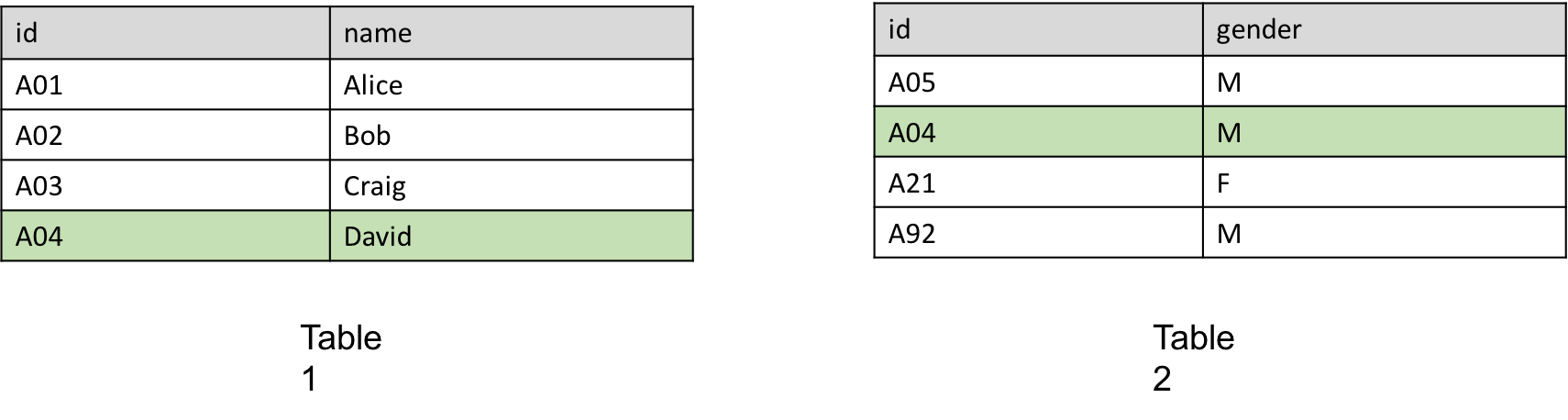It’s obvious that both of them have identical id A04 and these two belong to the same entity. Then we know that “David’s id is A04 and he is a male”.

But real world situations are more complex:

• Typos: “Joh” vs “John”
• OCR errors: “J0hn” vs “John”
• Formatting conventions: “03/17” vs “March 17”
• Abbreviations: “J. K. Rowling” vs “Joanne Rowling”
• Nick names: “John” vs “Jock”
• Word order: “Sargent, John S.” vs “John S. Sargent”

Record Linkage Toolkit (RLTK) is designed to give a easy to use, scalable and extensible way of resolving these problems.

## Basic Components & Data Flow¶

The first step of using RLTK is to create Dataset.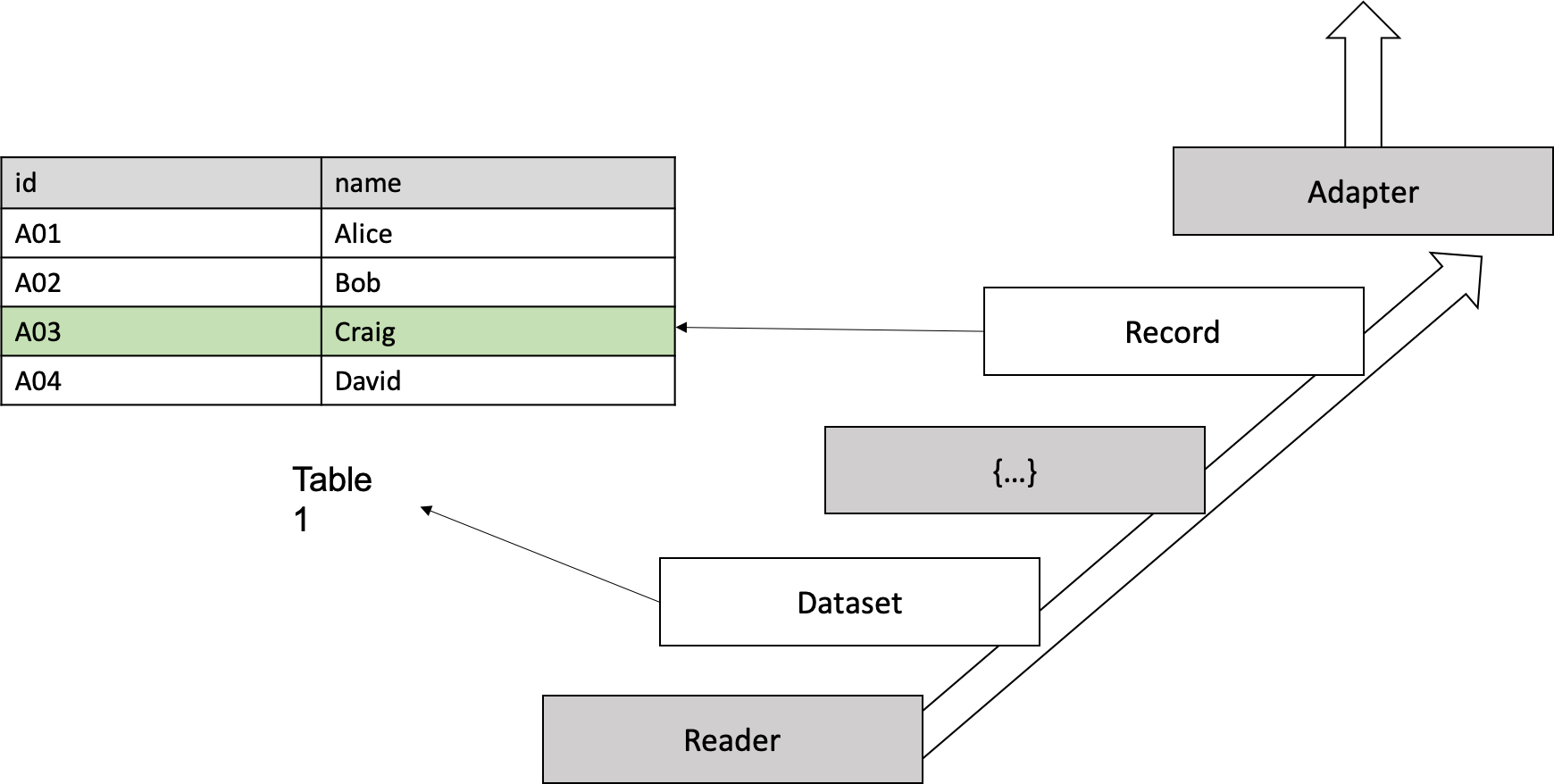In RLTK, every “row” in table is called Record. Notice “row” here is a logical concept: for a csv file it’s a csv object (can be formed by multiple lines); for a json lines file, it’s a line object; for a query from DBMS, it’s one row; for some self-defined stream, it’s a minimal entity.

Dataset is a “table”, or more precise, a collection of Record s.

Reader is used to handle heterogeneous input. For each “row”, the return of the Reader is represented in a Python dictionary, named raw_object (shown as grey {...} in figure). Record is generated based on raw_object: user need to extend base Record class and add properties for later usage.

KeyValueAdapter is where all Record s get stored. It can be either in memory or persistent.

So, the data flow is: in order to create Dataset, use Reader to read from input source and convert entity by entity to raw_object which is used to construct Record, then store Record in KeyValueAdapter.

Obviously, generating Record is really time consuming if the dataset is large, but if the concrete KeyValueAdapter is a persistent one (e.g., HBaseKeyValueAdapter), then next time, Dataset can be loaded directly from this KeyValueAdapter instead of regenerating again from raw input.

## Minimal Workflow & Implementation¶

Now we have two Datasets and we need to find pairs (If it’s de-duplication problem, only one Dataset is needed).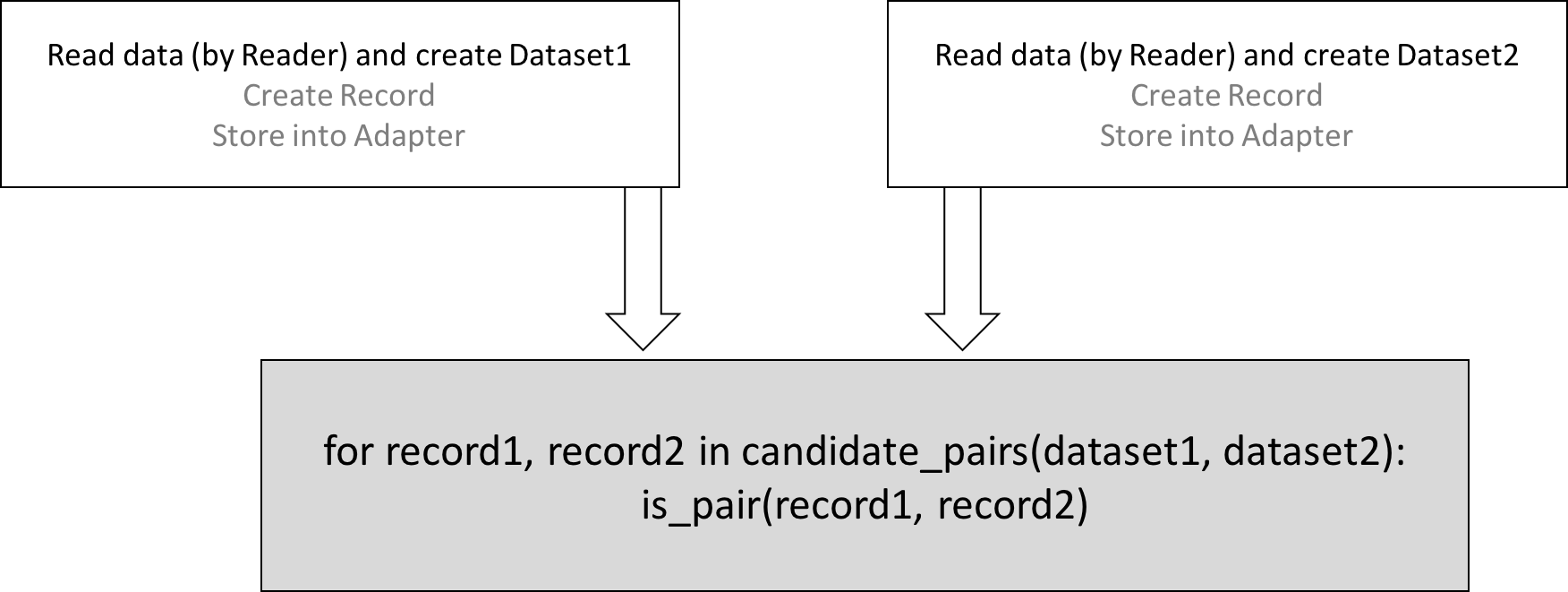Simply using RLTK’s API to get all possible combinations of candidate pairs and implement your only “magical function” (shown as is_pair() in figure) to find if two Record s are the same.

Let’s look at example input datasets and minimal implementation.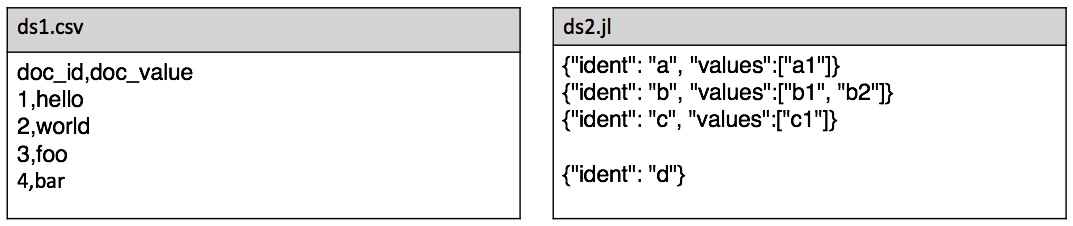import rltk

class Record1(rltk.Record):
@property
def id(self):
return self.raw_object['doc_id']

@property
def value(self):
return self.raw_object['doc_value']

class Record2(rltk.Record):
@rltk.cached_property
def id(self):
return self.raw_object['ident']

@rltk.cached_property
def value(self):
v = self.raw_object.get('values', list())
return v if len(v) > 0 else 'empty'

pairs = rltk.get_record_pairs(ds1, ds2)
for r1, r2 in pairs:
print('-------------')
print(r1.id, r1.value, '\t', r2.id, r2.value)
print('levenshtein_distance:', rltk.levenshtein_distance(r1.value, r2.value))
print('levenshtein_similarity:', rltk.levenshtein_similarity(r1.value, r2.value))


One thing to notice here: the property in Record class can be decorated by @property, or @rltk.cached_property which pre-calculates the value instead of computing at the runtime.

For the “magical function”, you can use any methods that make sense: hand-crafted rules, machine learning model, etc. RLTK provides a lot of similarity metrics which can be very helpful while doing comparison.

## Evaluation¶

After designing the “magical function”, you need a way to judge its performance. RLTK has a built-in package called Evaluation which includes three basic components:

• Groud Truth: Ground truth data.
• Trial: Store the result of prediction of candidate pairs.
• Evaluation: Visualize the result of evaluation if multiple trials are given.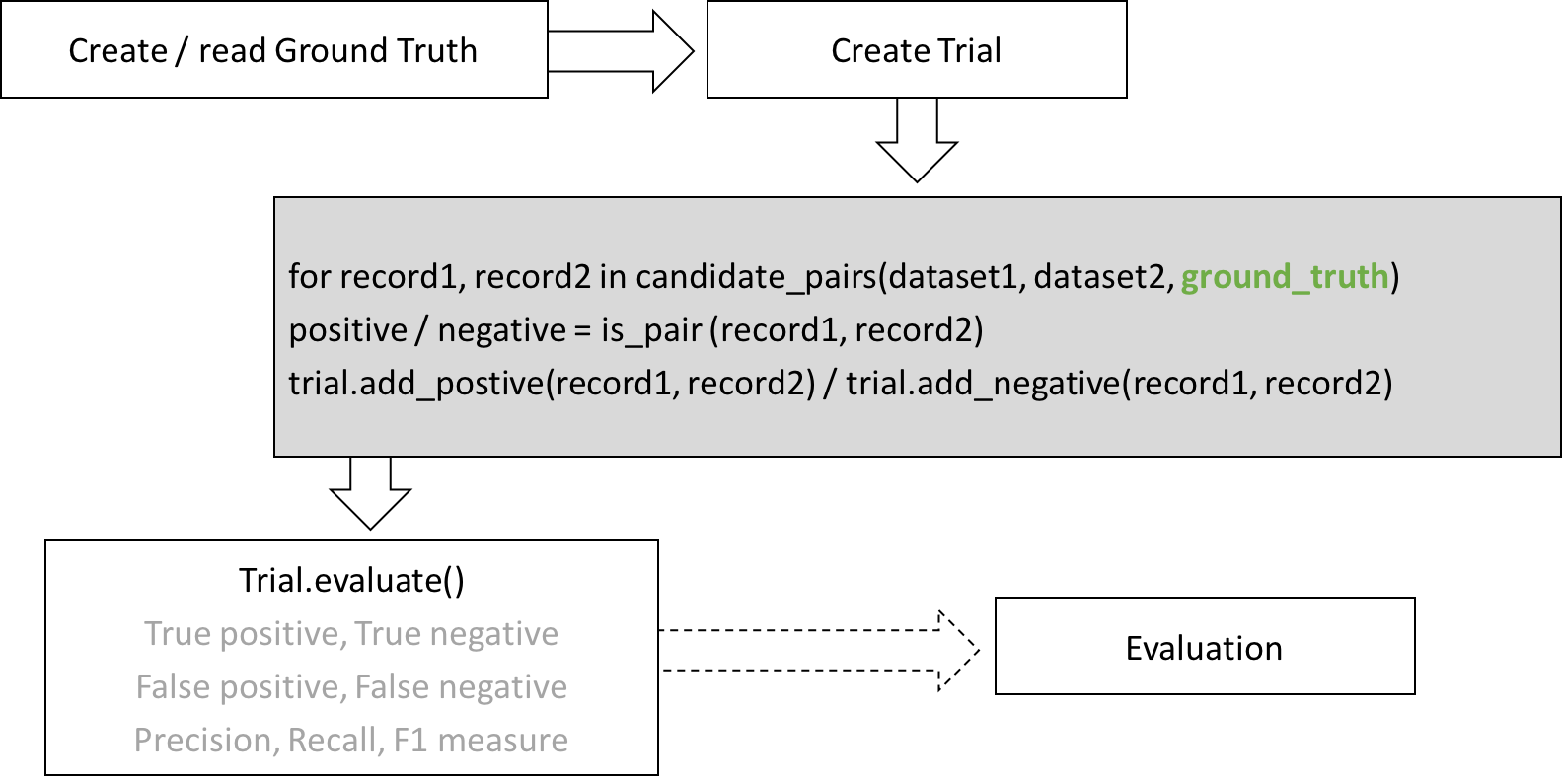As can be seen from the figure, every Trial has a corresponding GroundTruth. GroundTruth needs to be provided while generating candidate pairs. Add prediction result to Trial if it needs to be evaluated later. Call evaluate() to get the evaluation of the Trial against GroundTruth.

gt = rltk.GroundTruth()
eva = rltk.Evaluation()
trial = rltk.Trial(ground_truth=gt)

test_pairs = rltk.get_record_pairs(ds1, ds2, ground_truth=gt):
for r1, r2 in test_pairs:
is_positive = is_pair(r1, r2)

trial.evaluate()
print(trial.true_positives, trial.false_positives, trial.true_negatives, trial.false_negatives,
trial.precision, trial.recall, trial.f_measure)


Notice add_positive() and add_negative() are just syntactic sugar of add_result() used in above code snippet.

## Blocking¶

When finding pairs between two datasets, how many total comparison does it make?

Let’s say the 1st dataset has M items and and 2nd has N, then it needs M*N comparisons. If M=10,000, N=100,000, M*N=1,000,000,000. If the computer can determine a heavy is_pair() in 0.001s, in total it costs 1 billion x 0.001s / 60 / 60 / 24 = 11.57 days. Apparently exhausting is not a good choice. Blocking is something invented to tackle this problem. Blocking attempts to restrict comparisons to just those records for which one or more particularly discriminating identifiers agree, which has the effect of increasing the positive predictive value (precision) at the expense of sensitivity (recall).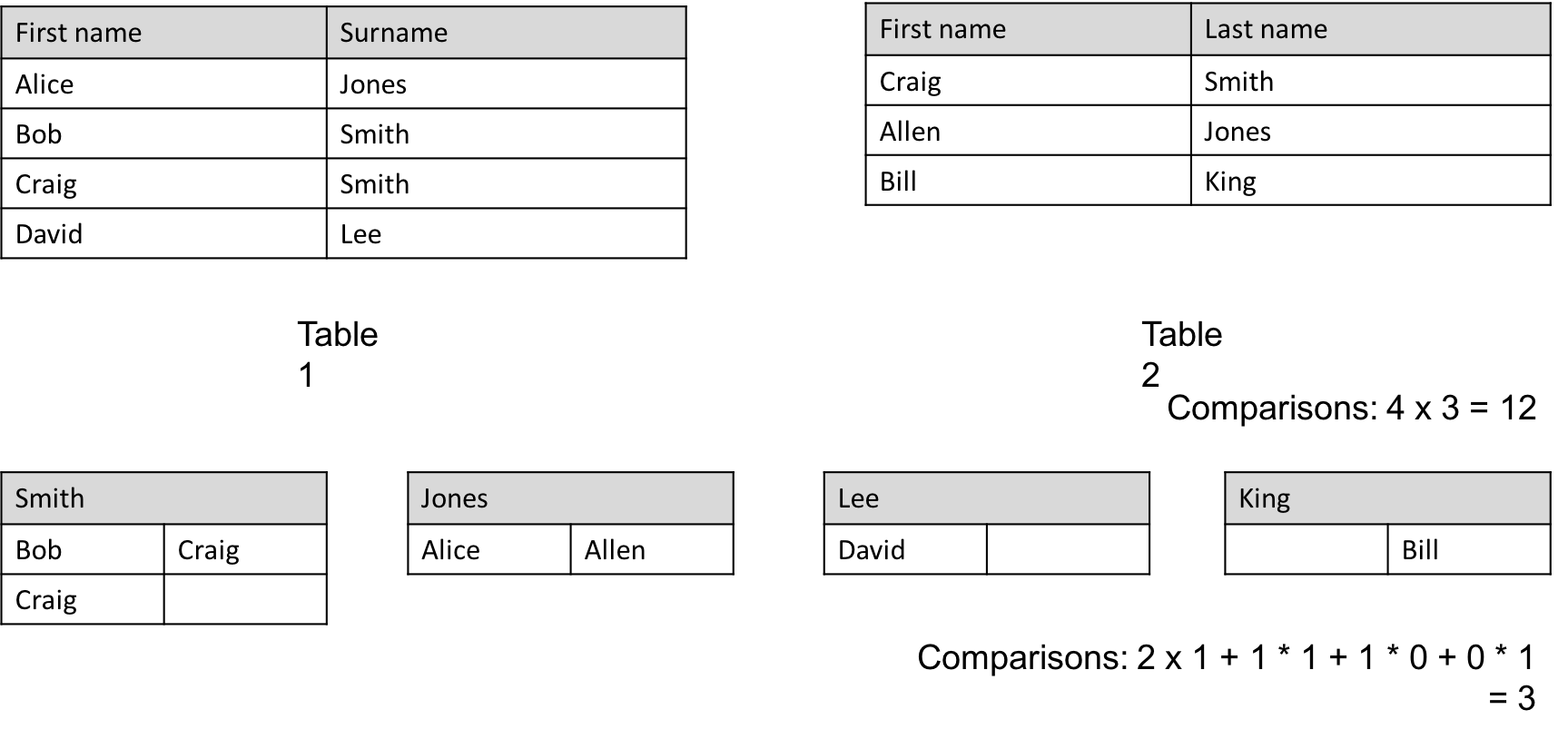For example: Full comparison (cross product) of two tables (shown in figure) is 12 times. After inspection, it’s obvious to say that “last name” can be used as blocking key (group by based on key) since people who have different last name can’t be the same. Then, total comparison drops to 3 times.

Blocks need to be calculated and passed while generating candidate pairs. Blocks’ calculation can be time consuming so RLTK supports dumping them to disk for further usage.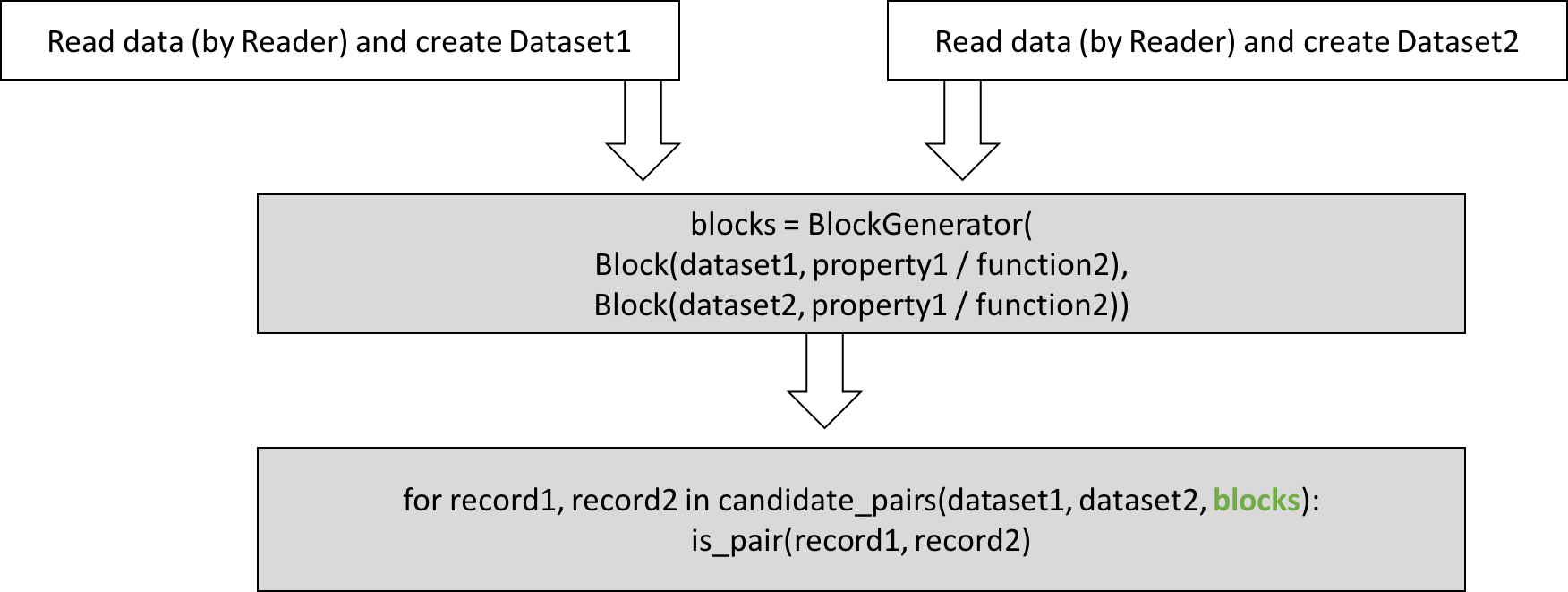def get_first_name(r2):
return r2.full_name.split(' ')

bg = rltk.HashBlockGenerator()
block = bg.generate(
bg.block(ds1, property_='first_name'),
bg.block(ds2, function_=get_first_name)),
pairs = rltk.get_record_pairs(ds1, ds2, block=block)
for r1, r2 in pairs:
print(r1.id, r1.full_name, '\t', r2.id, r2.full_name)


## Summary¶

Now you should know what’s the goal of record linkage, how to construct Dataset and how to use it in RLTK workflow, how to evaluate the quality of linkage and how to use blocking technique to deal with large datasets.